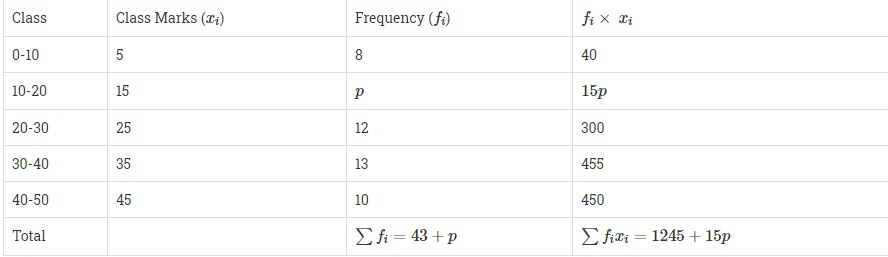# If the mean of the following distribution is 27, find the value of $p$.Class:0-810-2020-3030-4040-50Frequency:8$p$121310

Given:

The mean of the given distribution is 27.

To do:

We have to find the value of $p$.

Solution:

Mean $=27$We know that,

Mean $=\frac{\sum{f_ix_i}}{\sum{f_i}}$

Therefore,

Mean $27=\frac{1245+15p}{43+p}$

$27(43+p)=1245+15p$

$1161+27p=1245+15p$

$1245-1161=27p-15p$

$p=\frac{84}{12}$

$p=7$

The value of $p$ is $7$.